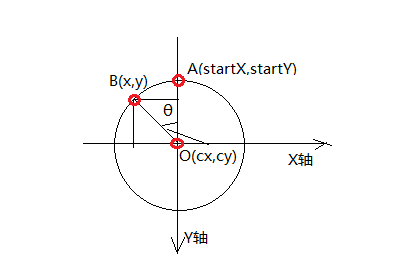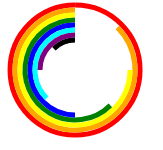# Javascript动态创建SVG圆弧

## 1. 问题描述1. 圆弧起点$A$$A$的坐标$\left(startX,startY\right)$$(startX,startY)$
2. 圆弧的半径 $R$$R$
3. 圆弧终点$B$$B$与起点$A$$A$构成的圆心角$\theta$$\theta$，单位为度
4. 圆弧线条的宽度 $width$$width$
5. 圆弧线条的颜色 $color$$color$

1. 圆弧起点$A$$A$为圆弧上$Y$$Y$坐标值最小的点（在屏幕坐标系中）
2. 只按逆时针方向绘制圆弧（起点到终点）

## 2. SVG中的path

SVG中的path元素可以用来绘复杂的图形。它的形状是通过属性d来定义的，属性d的值是”命令+参数”序列。有过在AutoCAD中使用命令来绘图的应该能很快理解。

• M x y
把画笔移动到点$\left(x,y\right)$$(x,y)$ (MMove的缩写)
• A rx ry x-axis-rotation large-arc-flag sweep-flag x y
按给定参数绘制圆弧，各部分参数的解释如下：
• rx ry
圆弧的$x$$x$轴半径和$y$$y$轴半径 A命令也可以用来绘制椭圆弧的，如果是圆弧则 $rx=ry$$rx=ry$
• x-axis-rotation
$x$$x$轴的旋转角度，这里我们用不到，设为 0
• large-arc-flag
是否为大圆弧，1为是，0为否
• sweep-flag
圆弧绘制的方向（从起点到终点），1为顺时针，0为逆时针
• x y
圆弧终点的坐标$\left(x,y\right)$$(x,y)$

### 2.1 分析

1. M命令来移动到圆弧的起点$A\left(startX,startY\right)$$A(startX,startY)$，即 M startX startY
2. 圆弧的半径rxry可以从已知条件$R$$R$中求得
3. $x$$x$轴的旋转角度 x-axis-rotation，我们不管这个参数，这里设为0
4. 是否为大圆弧 large-arc-flag 这个参数，我们可以根据$\theta$$\theta$这个参数计算得出，如果$\theta >=180$$\theta>=180$，则为大圆弧，反之为小圆弧
5. 圆弧绘制的方向 sweep-flag问题描述假设部分规定绘制方向为逆时针，所以该值为0
6. 线条宽度，对应pathstroke-width属性
7. 线条颜色，对应pathstroke属性
8. pathfill属性应设为none，即无填充

$\begin{array}{}\text{(1)}& \left\{\begin{array}{lr}x=cx+R\mathrm{cos}\left(90+\theta \right)& \\ y=cy-R\mathrm{sin}\left(90+\theta \right)\end{array}\end{array}$

$\alpha =\frac{\theta }{180}\pi$

### 2.2 注意点

SVG中，对于圆弧而言，线条的宽度不包含在半径中，线条宽度是向外延伸的。因此在后续参数的计算中需要考虑到这一点，相关参数需要根据线条宽度来调节。

## 3.编码实现

function createSVGPath(startX, startY, R, theta, width, color) {
var path = document.createElementNS('http://www.w3.org/2000/svg', 'path');
var realR = R - width;
var dArr = ["M" + startX, startY + width, "A" + realR, realR, 0, theta>=180 ? 1 : 0, 0];
var cx = startX, cy = startY + R;

var theta2 = theta%360;
// 避免360度与0度一样的情况
theta = theta > 0 && theta2 == 0 ? 359.9 : theta2;

var alpha = (theta + 90)/180 * Math.PI;
var dx = realR * Math.cos(alpha);
var dy = realR * Math.sin(alpha);
var x = cx + dx, y = cy - dy;

dArr.push(x.toFixed(2));
dArr.push(y.toFixed(2));
var d = dArr.join(" ");

path.setAttribute('d', d);
path.setAttribute('stroke', color);
path.setAttribute('stroke-width', width);
path.setAttribute('fill', 'none');

return path;
}

## 4. 测试

<!DOCTYPE html>
<html>
<head>
<meta charset="utf8">
<title>动态生成SVG圆弧测试</title>
</head>
<body>
<svg xmlns="http://www.w3.org/2000/svg" version="1.1" height="150px" width="150px" id="svg-01">
</svg>
</body>
<script>
function createSVGPath(startX, startY, R, theta, width, color) {
var path = document.createElementNS('http://www.w3.org/2000/svg', 'path');
var realR = R - width;
var dArr = ["M" + startX, startY + width, "A" + realR, realR, 0, theta>=180 ? 1 : 0, 0];
var cx = startX, cy = startY + R;

var theta2 = theta%360;
// 避免360度与0度一样的情况
theta = theta > 0 && theta2 == 0 ? 359.9 : theta2;

var alpha = (theta + 90)/180 * Math.PI;
var dx = realR * Math.cos(alpha);
var dy = realR * Math.sin(alpha);
var x = cx + dx, y = cy - dy;

dArr.push(x.toFixed(2));
dArr.push(y.toFixed(2));
var d = dArr.join(" ");

path.setAttribute('d', d);
path.setAttribute('stroke', color);
path.setAttribute('stroke-width', width);
path.setAttribute('fill', 'none');

return path;
}

var svg = document.getElementById('svg-01');
var colors = ['red', 'orange', 'yellow', 'green', 'blue', 'cyan', 'purple', 'black'];
for(var i=0; i<8; i++){
var startY = i*5;
var R = 70 - startY;
var theta = 360 - 45* i;
var path = createSVGPath(75, startY, R, theta, 5, colors[i]);
svg.appendChild(path);
}
</script>
</html>